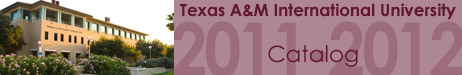Home >> Course Descriptions >> College of Arts and Sciences MATH 4310 Abstract Algebra I Three semester hours. (SP) Introduction to abstract algebra. Topics include Introduction to Ring theory: arithmetic in integers, modular arithmetic, fields, arithmetic in the Ring of polynomials. Unique factorization in integers and polynomials. Introduction to Group theory: definition and examples, subgroups, quotient groups, symmetry groups and permutation groups. Prerequisite: MATH 3365.